# Electronics and Communication Engineering - Digital Electronics

36.

Logic analyser is

 A. a multichannel oscilloscope B. similar to logic pulser C. similar to current tracer D. none of the above

Explanation:

It is a multichannel oscilloscope.

37.

The minimized version of logic circuit in the given figure is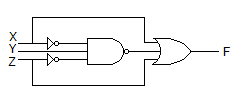A.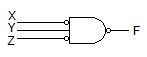B.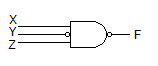C.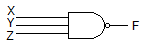D.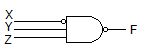Explanation:

The Boolean equation is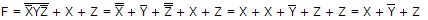The circuit in the given figuregives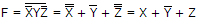.

38.

In the given figure shows a negative logic AND gate. If positive logic is used this gate is equivalent to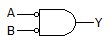A. AND gate B. OR gate C. NOR gate D. NAND gate

Explanation:

Y = A B = A + B .

39.

The function Y = A B C + AB C + A B C + A BC is to be realized using discrete gates. The inputs available are A, B, C. We need a total of

 A. 8 gates B. 6 gates C. 10 gates D. 5 gates

Explanation:

Three NOT gates, four AND gates and one OR gate, i.e., total of 8 gates.

40.

A 10 bit D/A converter gives a maximum output of 10.23 V. The resolution is

 A. 10 mV B. 20 mV C. 15 mV D. 25 mV

Resolution =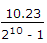V or 10 mV.